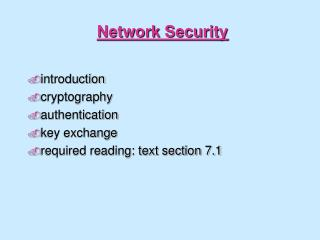DownloadDownload PresentationNetwork Security

# Network Security

Download Presentation## Network Security

- - - - - - - - - - - - - - - - - - - - - - - - - - - E N D - - - - - - - - - - - - - - - - - - - - - - - - - - -
##### Presentation Transcript

1. Network Security • introduction • cryptography • authentication • key exchange • required reading: text section 7.1

2. Network Security Intruder may • eavesdrop • remove, modify, and/or insert messages • read and playback messages

3. Important issues: • cryptography: secrecy of info being transmitted • authentication: proving who you are and having correspondent prove his/her/its identity

4. Security in Computer Networks User resources: • login passwords often transmitted unencrypted in TCP packets between applications (e.g., telnet, ftp) • passwords provide little protection

5. Network resources: • often completely unprotected from intruder eavesdropping, injection of false messages • mail spoofs, router updates, ICMP messages, network management messages Bottom line: • intruder attaching his/her machine (access to OS code, root privileges) onto network can override many system-provided security measures • users must take a more active role

6. Encryption plaintext: unencrypted message ciphertext: encrypted form of message Intruder may • intercept ciphertext transmission • intercept plaintext/ciphertext pairs • obtain encryption decryption algorithms

7. A simple encryption algorithm Substitution cipher: abcdefghijklmnopqrstuvwxyz poiuytrewqasdfghjklmnbvczx • replace each plaintext character inmessage with matching ciphertext character: plaintext:Charlotte, my love ciphertext:iepksgmmy, dz sgby

8. key is pairing between plaintext characters and ciphertext characters • symmetric key: sender and receiver use same key • 26! (approx 10^26) different possible keys: unlikely to be broken by random trials • substitution cipher subject to decryption using observed frequency of letters • 'e' most common letter, 'the' most common word

9. DES: Data Encryption Standard • encrypts data in 64-bit chunks • encryption/decryption algorithm is a published standard • everyone knows how to do it • substitution cipher over 64-bit chunks: 56-bit key determines which of 56! substitution ciphers used • substitution: 19 stages of transformations, 16 involving functions of key

10. decryption done by reversing encryption steps • sender and receiver must use same key

11. Key Distribution Problem Problem: how do communicant agree on symmetric key? • N communicants implies N keys Trusted agent distribution: • keys distributed by centralized trusted agent • any communicant need only know key to communicate with trusted agent • for communication between i and j, trusted agent will provide a key

12. We will cover in more detail shortly

13. Public Key Cryptography • separate encryption/decryption keys • receiver makes known (!) its encryption key • receiver keeps its decryption key secret • to send to receiver B, encrypt message M using B's publicly available key, EB • send EB(M) • to decrypt, B applies its private decrypt key DB to receiver message: • computing DB( EB(M) ) gives M

14. knowing encryption key does not help with decryption; decryption is a non-trivial inverse of encryption • only receiver can decrypt message Question: good encryption/decryption algorithms

15. RSA: public key encryption/decryption RSA: a public key algorithm for encrypting/decrypting entity wanting to receive encrypted messages: • choose two prime numbers, p, q greater than 10^100 • compute n=pq and z = (p-1)(q-1) • choose number d which has no common factors with z • compute e such that ed = 1 mod z, i.e., integer-remainder( (ed) / ((p-1)(q-1)) ) = 1, i.e., ed = k(p-1)(q-1) +1 • three numbers: • e, n made public • d kept secret

16. RSA (continued) to encrypt: • divide message into i blocks, bi of size k: 2**k < n • encrypt: encrypt(bi) = bi**e mod n to decrypt: • bi = encrypt(bi)**d to break RSA: • need to know p, q, given pq=n, n known • factoring 200 digit n into primes takes 4 billion years using known methods

17. RSA example • choose p=3, q=11, gives n=33, (p-1)(q-1)=z=20 • choose d = 7 since 7 and 20 have no common factors • compute e = 3, so that ed = k(p-1)(q-1)+1 (note: k=1 here)

18. Further notes on RSA why does RSA work? • crucial number theory result: of p, q prime then bi**((p-1)(q-1)) mod pq = 1 • using mod pq arithmetic: (b**e)**d = b**(ed) = b**(k(p-1)(q-1)+1) for some k = b b**(p-1)(q-1) b**(p-1)(q-1) ... b**(p-1)(q-1) = b 1 1 ... 1 = b Note: we can also encrypt with d and encrypt with e. • this will be useful shortly

19. How to break RSA? Brute force: get B's public key • for each possible bi in plaintext, compute bi**e • for each observed bi**e, we then know bi • more: choose size of bi "big enough"

20. man-in-the-middle: intercept keys, spoof identity: